Letter to Entering Biochemistry Students

Question: Why should a biochemist care about calculus and physics?

1) The crude answer: On average, grades in senior-level physical chemistry are directly related to grades previously earned in math and physics classes.

Many students find physical chemistry difficult not because the concepts are hard, but because they have to apply the calculus and physics they learned previously to problems in chemistry. When freshmen and sophomores merely memorize calculus and physics for their exams rather than truly learning the material, they have difficulty later in senior-level physical chemistry courses. Of course, lack of preparation can be overcome by extraordinary personal effort, but it is easier to put in a little effort now rather than a lot of effort later.

2) The subtle answer: By understanding physical chemistry, a student will have the skills to quantitatively understand biochemical processes. In order to understand physical chemistry, a student must first understand math and physics.

Physical chemistry is useful to biochemists because they use concepts including energies of reactions, probabilities of reactions, entropy, heat, phase transitions, chemical potential, and electrochemistry. These concepts are not only useful (particularly in scientific research), but beautiful as well! If students plan careers that use their biochemistry knowledge and the concepts above, then they should pay attention in their physical chemistry, math, and physics courses.

Data demonstrating that higher grades in math and physics translate into higher grades in physical chemistry for biochemists (Chem452 in 2001):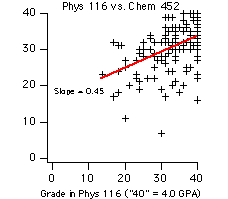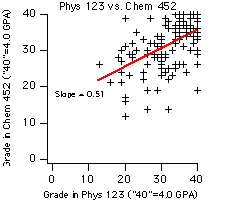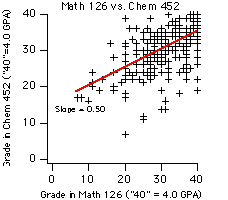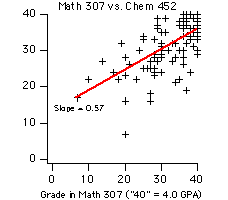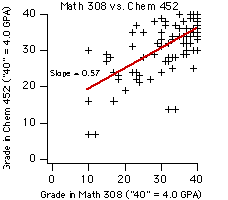Good Resources:

Other than standard math textbooks, there are some specialized materials to help chemists and biochemists review the necessary mathematics. Books that have sections useful to Chem452 and Chem453 in particular are listed below:

(1) Applied Mathematics for Physical Chemistry, James Barrante, Prentice Hall Publishers

- Section 3 on logs is useful.

- Section 4 on differential calculus is worth skimming.

- Section 5 on integrals is useful.

(2) Mathematics for Physical Chemistry, Robert G. Mortimer, Macmillan Press

- The discussion of calculus with several independent variables at the beginning of Chapter 6 is very useful.

(3) To prepare for Chem453, see Principles of Physical Chemistry, Lionel M. Raff, Prentice Hall

- Chapter 10 on "the mathematics of chance" is useful.

Compiled by Sarah L. Keller